# 感知机算法原理（PLA原理）及 Python 实现

PLAPerceptron Learning Algorithm 的缩写，也叫感知机算法。感知机算法与线性回归有很多相似的地方。例如线性回归采用梯度下降法求最优参数的时候对每个数据点都进行了遍历，求误差的平均值。而 PLA 只选取一个点进行处理，所以 PLA 的学习过程也可以叫做 随机梯度下降

# 1 感知机模型

## 1.1 形象的感知机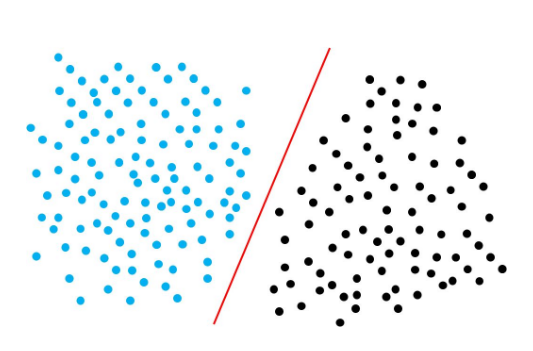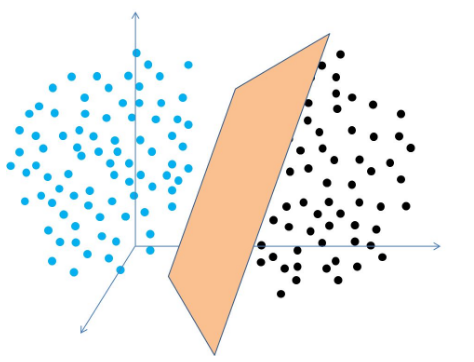## 1.2 感知机的数学表示

f(x)=sign(ωx+b)

sign(x)=+1,1,x0x<0

## 1.3 感知机的假设空间

H={f|f(x)=sign(ωx+b)}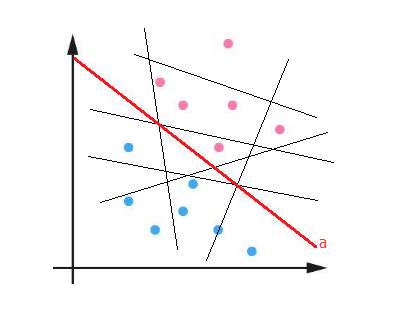## 1.4 感知机的几何意义

ωx+b=0

1. 方程 ωx+b=0 $\omega\cdot x+b=0$ 是一个超平面的方程
2. ω $\omega$ 是这个超平面的法向量
3. b $b$ 是这个超平面的截距## 1.5 感知机的输入和输出

H $\mathcal{H}$假设空间
f(x)H $f(x)\in \mathcal{H}$感知机模型
XRn $\mathcal{X}\subseteq R^n$特征空间
Y={+1,1} $\mathcal{Y}=\{+1,-1\}$输出空间
Rn $R^n$ n $n$ 维向量空间
ω=(ω(1),ω(2),...,ω(n))$\omega=(\omega^{(1)},\omega^{(2)},...,\omega^{(n)})$超平面的法向量，或者说特征的参数向量
ω(i),(i=1,2,...,n) $\omega^{(i)},(i=1,2,...,n)$超平面的法向量的分量，或者说某个参数
x=(x(1),x(2),...,x(n)) $x=(x^{(1)},x^{(2)},...,x^{(n)})$输入向量，特征向量
x(i),(i=1,2,...,n) $x^{(i)},(i=1,2,...,n)$特征向量中的某个特征
b $b$ 超平面的截距
sign(x)$sign(x)$符号函数, x0 $x\geqslant 0$ 时为 +1 $+1$，否则为 1 $-1$
D=(x1,y1),(x2,y2),...,(xm,ym) $\mathcal{D}={(x_1,y_1),(x_2,y_2),...,(x_m,y_m)}$数据集， m $m$ 为样本数, n$n$ 为特征数， y $y$ 为分类标签（1或者-1）

D=x1,x2,...,xm=x(1)1x(1)2x(1)mx(2)1x(2)2x(2)mx(n)1x(n)2x(n)my1y2ym

# 2. 感知机的学习过程

## 2.1 数据集的线性可分和线性不可分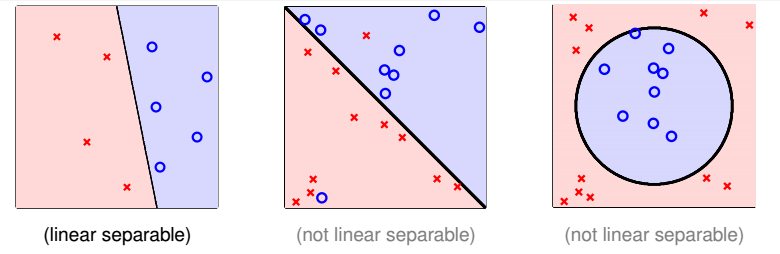D=(x1,y1),(x2,y2),...,(xn,yn)

ωx+b=0

## 2.2 PLA 原理及过程

PLA 的原理很简单，开始时，我们令 ω=b=0 $\omega=b=0$，然后在训练数据 D $\mathcal{D}$ 中任意选择一点，如果该点被错误分类了，那么我们就调整分类的直线或者超平面使该点能够被正确分类。

ab=i=1na(i)b(i)=abcosθ

ω^=(b,ω)=(ω0,ω)=(ω(0),ω(1),ω(2),...,ω(n)) $\hat \omega = (b,\omega) = (\omega^{0},\omega)=(\omega^{(0)},\omega^{(1)},\omega^{(2)},...,\omega^{(n)})$
x^=(1,x)=(1,x(1),x(2),...,x(n)) $\hat x = (1,x)=(1,x^{(1)},x^{(2)},...,x^{(n)})$

1. 输入数据 D=(x1,y1),(x2,y2),...,(xm,ym) $\mathcal{D}={(x_1,y_1),(x_2,y_2),...,(x_m,y_m)}$，其中 xiXRn $x_i\in \mathcal{X} \subset R^n$ yY={+1,1} $y\in \mathcal{Y} = \{+1,-1\}$ i=1,2,...,n $i=1,2,...,n$
2. 选取初值 ω^0=0 $\hat \omega_0 = 0$，即设为零向量。
3. 遍历 D $\mathcal{D}$ 中的数据，如果遇到某个样本 (xi,yi) $(x_i,y_i)$ 使得 yi(ω^x)0 $y_i(\hat \omega \cdot x) \leqslant 0$，即目前分类输出和真实分类不同，则
ω^ω^0+yix^i
4. ω^ $\hat \omega$ 更新后，回到第三步，重新开始遍历，如果遍历完整个数据集 D $\mathcal{D}$ 都未有更新操作（没有错误分类点），则转第五步。
5. 输出当前超平面的法向量 ω^ $\hat \omega$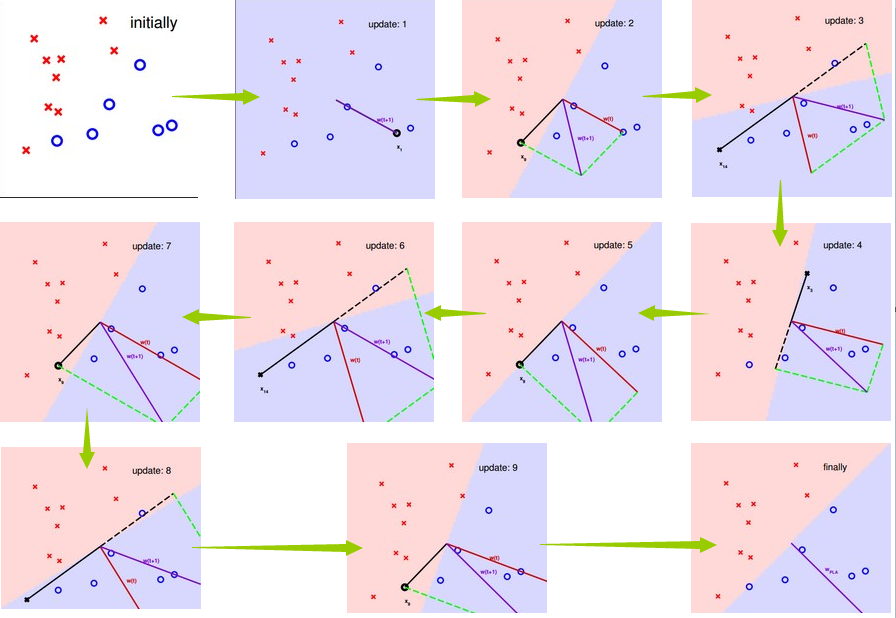## 2.3 PLA 的收敛性证明

γ=min0imyi(ω^x^i) $\gamma = \min\limits_{0\leqslant i \leqslant m}{y_i(\hat\omega\cdot\hat x_i)}$，即 γ $\gamma$ 为离超平面最近的数据点与超平面的距离（ m $m$ 为样本数量，后文中的含义相同）。那么，对于任意的点 x^i$\hat x_i$ 均有

yi(ω^x^i)γ

yi(ω^k1x^i)0

(x^i,yi) $(\hat x_i,y_i)$ 是被 ω^k1 $\hat\omega_{k-1}$ 错误分类的数据，则更新参数向量：

ω^k=ω^k1+yix^i

（1） ω^kω^optkγ $\hat\omega_k\cdot\hat\omega_{opt}\geqslant k\gamma$

ω^kω^opt=ω^k1^ωopt+yix^iω^optω^k1ωopt+γ

ω^k1ω^opt=ω^k2^ωopt+yix^iω^optω^k2ωopt+γ

ω^kω^optω^k1ωopt+γω^k2ωopt+2γ...ω^1ωopt+(k1)γω^0ωopt+kγ=kγ

（2） ω^k2kR2 ${\Vert\hat\omega_{k}\Vert}^2\leqslant kR^2$

ω^k2=ω^k12+2yiω^k1x^i+y2ix^i2,(yi{+1,1}y2i)

ω^k2ω^k12+x^i2

R=max0imx^i $R=\max\limits_{0\leqslant i \leqslant m}{\Vert\hat x_i\Vert}$，即离原点最远的点与原点的距离。则：
ω^k2ω^k12+R2

ω^k12ω^k22+R2

ω^k2ω^k12+R2ω^k22+2R2...ω^12+(k1)R2ω^02+kR2kR2

1cosθ=ω^kω^optω^kω^optkγω^kω^optkγkR2ω^opt

kR2ω^opt2γ2

# 3. 用 Python 实现感知机05-2252603-21316
10-29916
10-10775
01-06733
09-21455
06-3037
04-211万+
05-291万+
04-15104